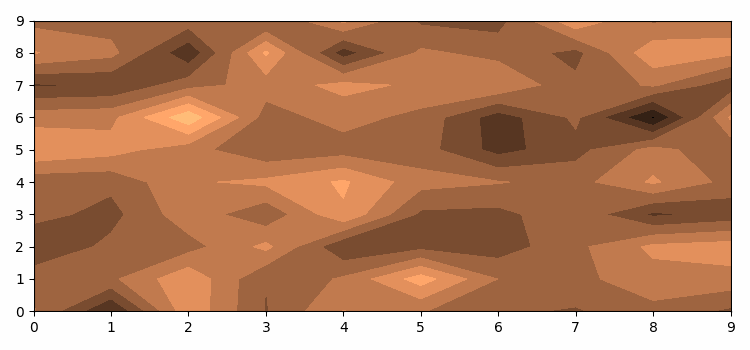# How do I display real-time graphs in a simple UI for a Python program?

MatplotlibPythonData Visualization

To display real-time graphs in a simple UI for a Python program, we can animate the contour plot.

## Steps

• Set the figure size and adjust the padding between and around the subplots.

• Create a random data of shape 10×10 dimension.

• Create a figure and a set of subplots using subplots() method.

• Make an animation by repeatedly calling a function *func*, using FuncAnimation() class.

• To update the contour value in a function, we can define a method, animate(), that can be used in FuncAnimation() class.

• To display the figure, use show() method.

## Example

import numpy as np
import matplotlib.pyplot as plt
import matplotlib.animation as animation

plt.rcParams["figure.figsize"] = [7.50, 3.50]
plt.rcParams["figure.autolayout"] = True
data = np.random.randn(800).reshape(10, 10, 8)
fig, ax = plt.subplots()

def animate(i):
ax.clear()
ax.contourf(data[:, :, i], cmap="copper")

ani = animation.FuncAnimation(fig, animate, 5, interval=100, blit=False)
plt.show()

## Output